Скачать презентацию Perfectly Sliced Every Time Graphics used with permission

38ce413975056fe44be5b8ac3cd8c8bc.ppt

• Количество слайдов: 38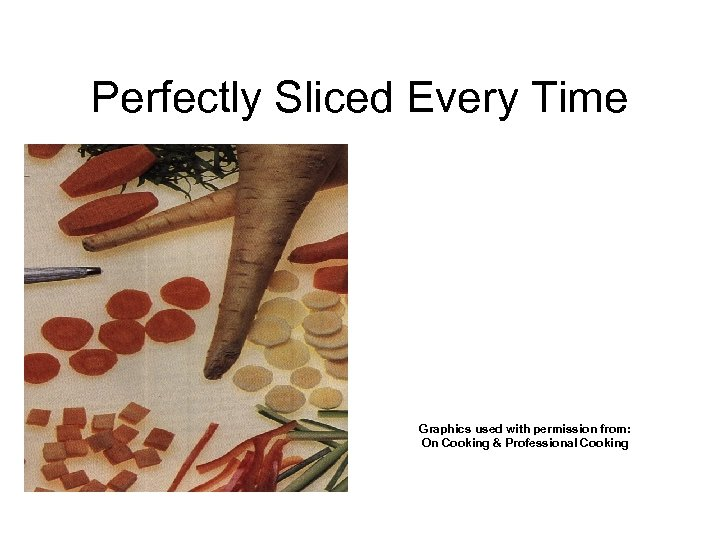Perfectly Sliced Every Time Graphics used with permission from: On Cooking & Professional Cooking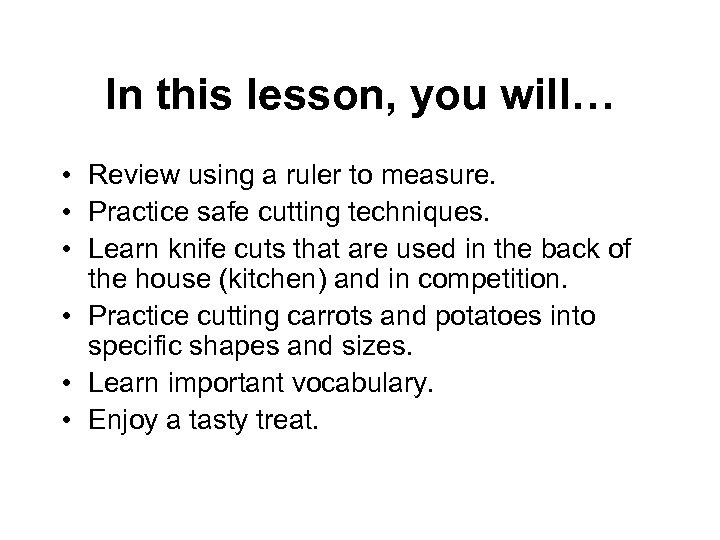In this lesson, you will… • Review using a ruler to measure. • Practice safe cutting techniques. • Learn knife cuts that are used in the back of the house (kitchen) and in competition. • Practice cutting carrots and potatoes into specific shapes and sizes. • Learn important vocabulary. • Enjoy a tasty treat.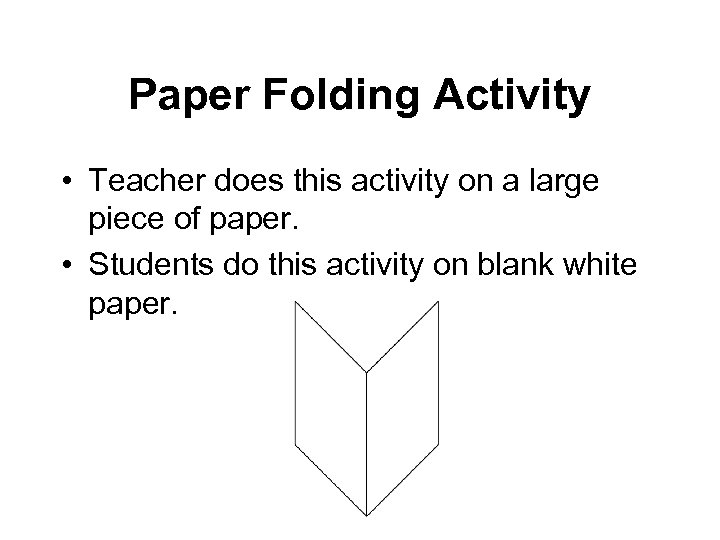Paper Folding Activity • Teacher does this activity on a large piece of paper. • Students do this activity on blank white paper.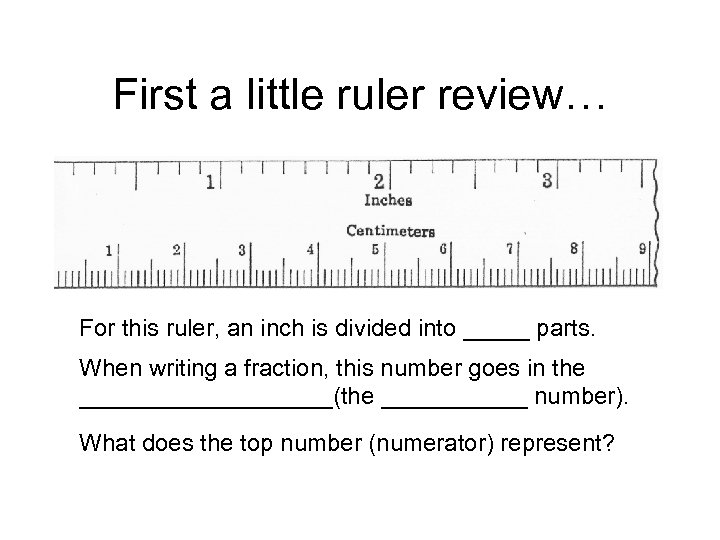First a little ruler review… For this ruler, an inch is divided into _____ parts. When writing a fraction, this number goes in the __________(the ______ number). What does the top number (numerator) represent?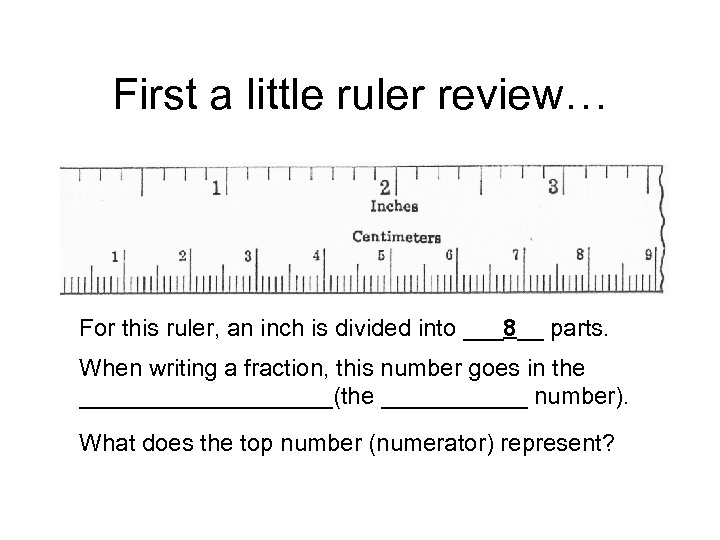First a little ruler review… For this ruler, an inch is divided into ___8__ parts. When writing a fraction, this number goes in the __________(the ______ number). What does the top number (numerator) represent?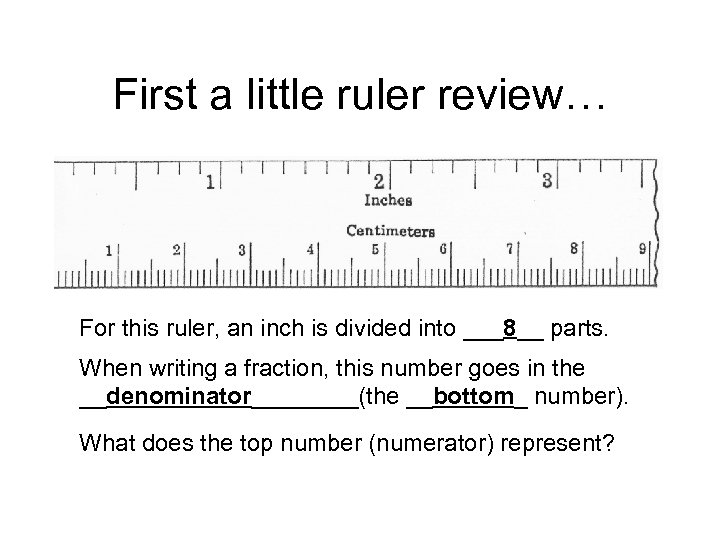First a little ruler review… For this ruler, an inch is divided into ___8__ parts. When writing a fraction, this number goes in the __denominator____(the __bottom_ number). What does the top number (numerator) represent?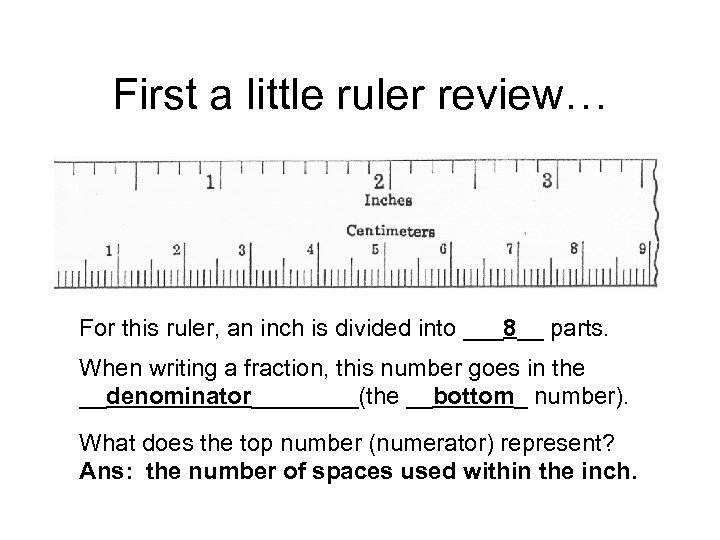First a little ruler review… For this ruler, an inch is divided into ___8__ parts. When writing a fraction, this number goes in the __denominator____(the __bottom_ number). What does the top number (numerator) represent? Ans: the number of spaces used within the inch.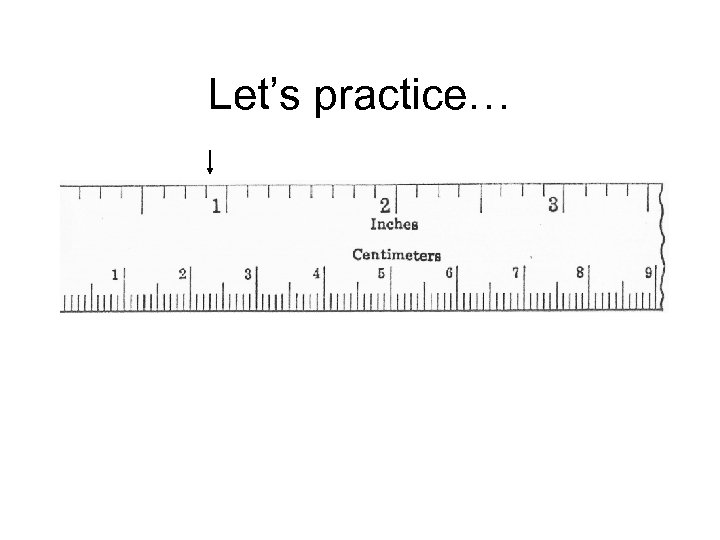Let’s practice…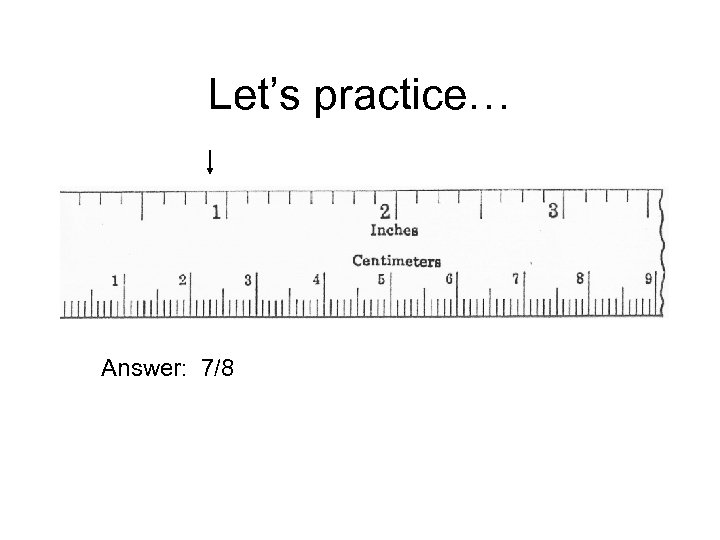Let’s practice… Answer: 7/8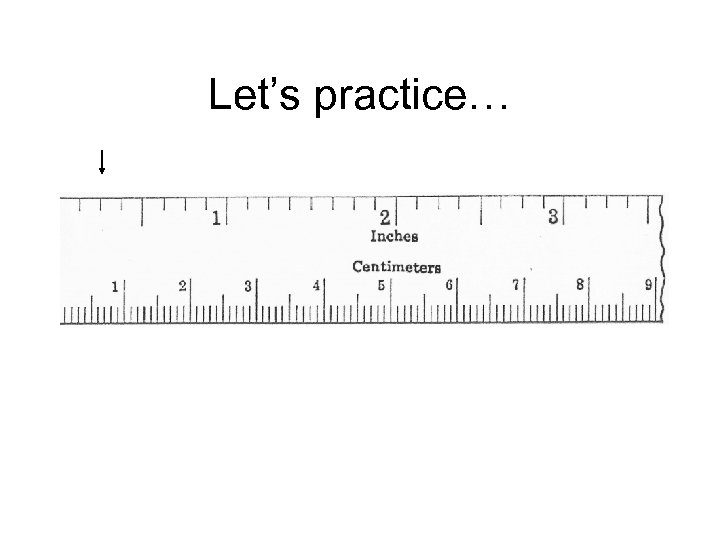Let’s practice…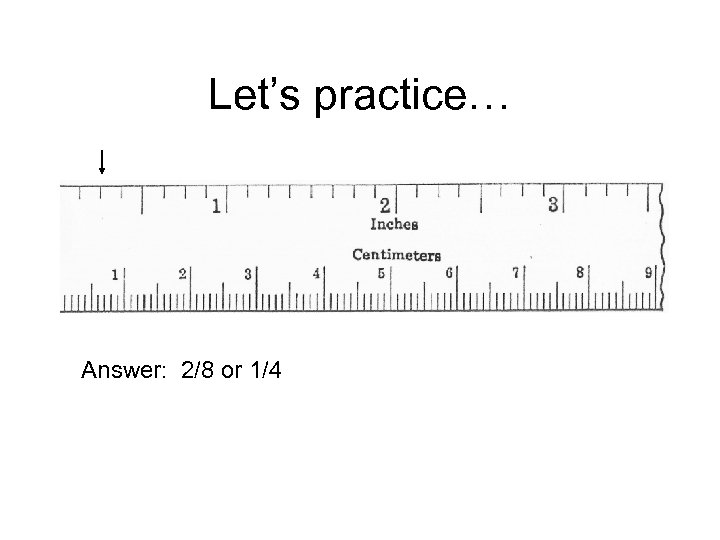Let’s practice… Answer: 2/8 or 1/4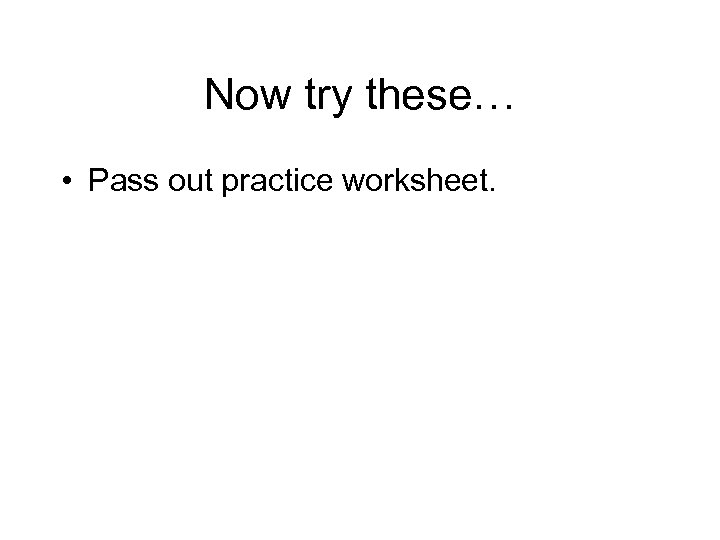Now try these… • Pass out practice worksheet.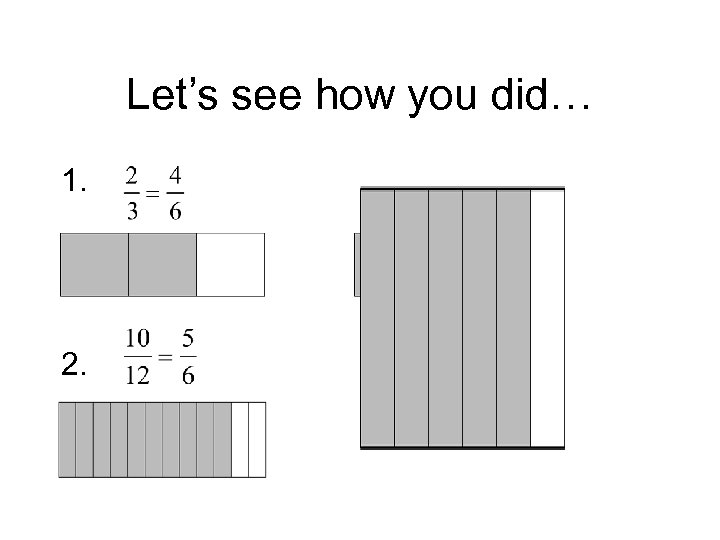Let’s see how you did… 1. 2.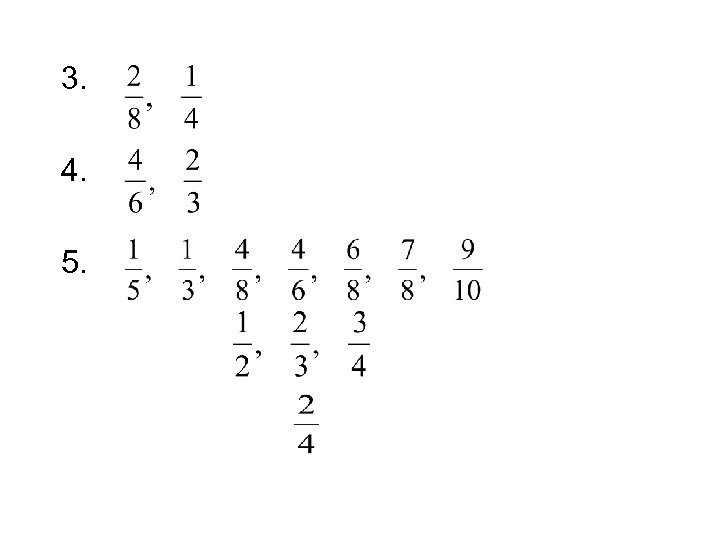3. 4. 5.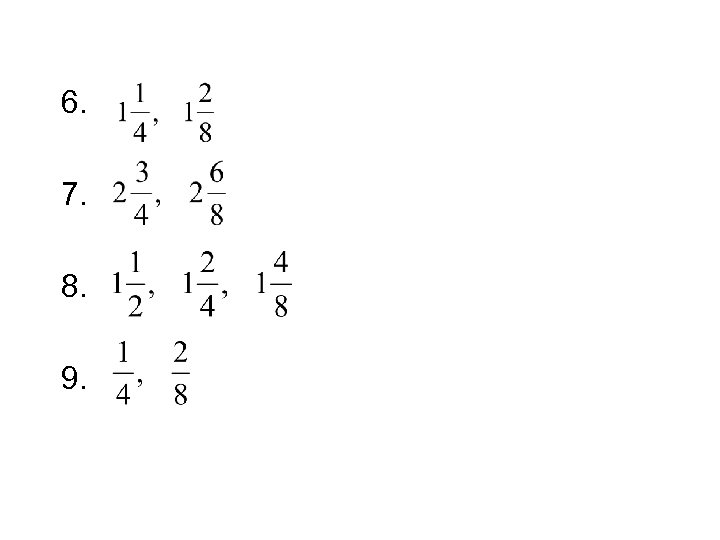6. 7. 8. 9.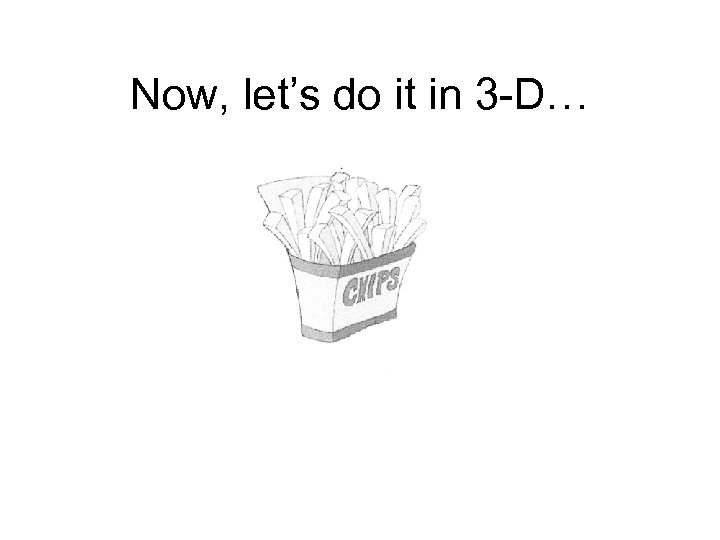Now, let’s do it in 3 -D…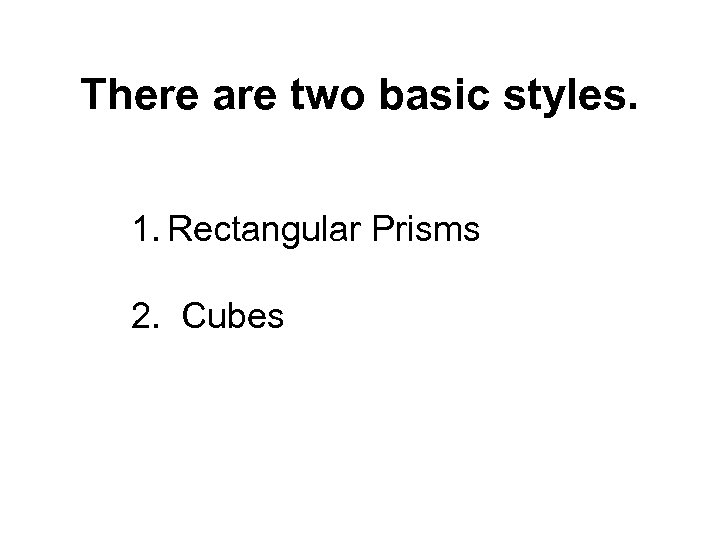There are two basic styles. 1. Rectangular Prisms 2. Cubes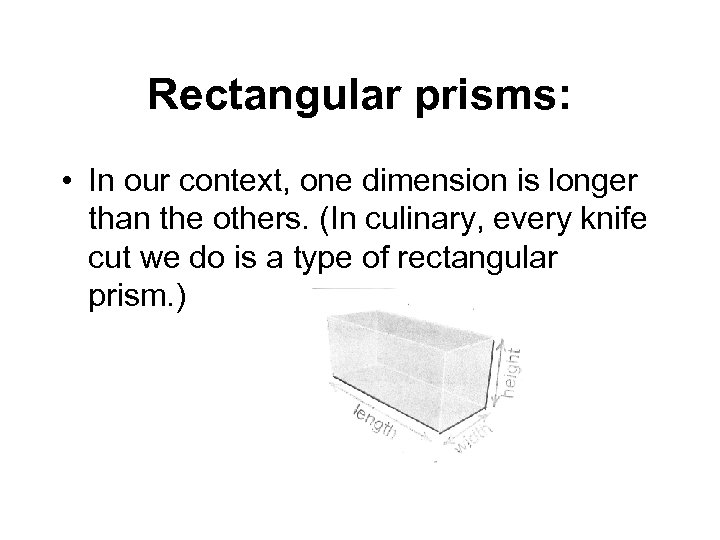Rectangular prisms: • In our context, one dimension is longer than the others. (In culinary, every knife cut we do is a type of rectangular prism. )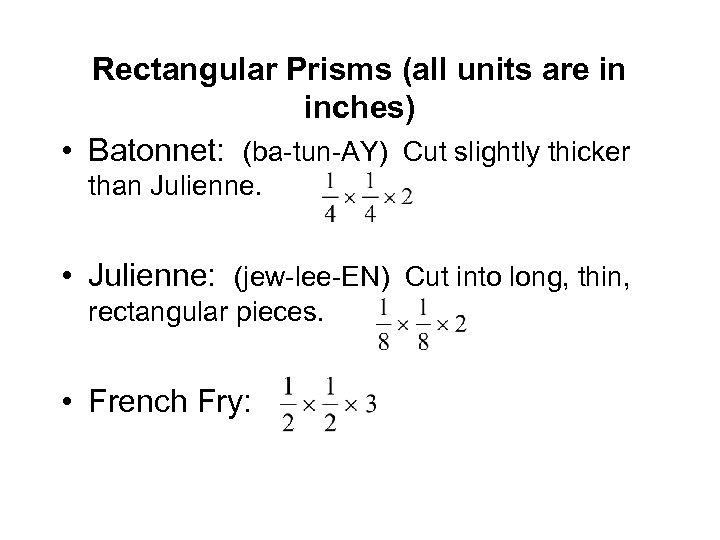Rectangular Prisms (all units are in inches) • Batonnet: (ba-tun-AY) Cut slightly thicker than Julienne. • Julienne: (jew-lee-EN) Cut into long, thin, rectangular pieces. • French Fry: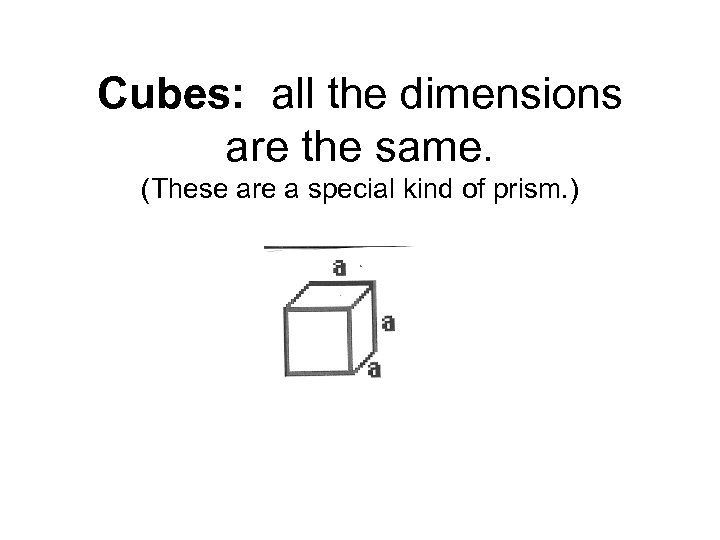Cubes: all the dimensions are the same. (These are a special kind of prism. )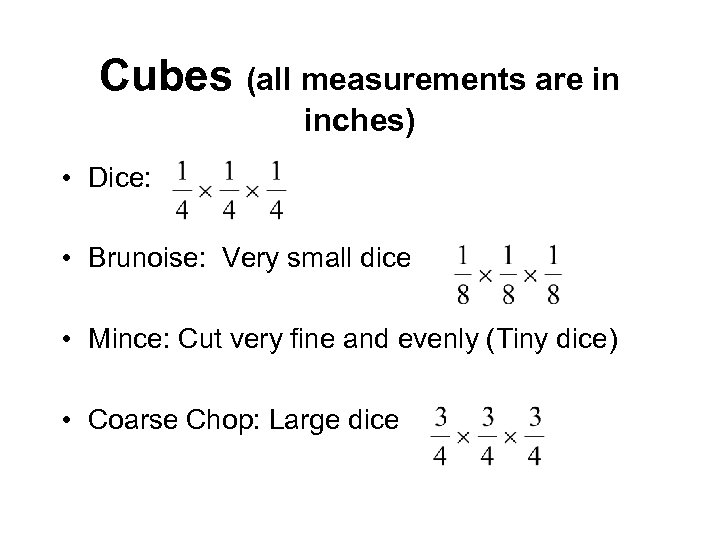Cubes (all measurements are in inches) • Dice: • Brunoise: Very small dice • Mince: Cut very fine and evenly (Tiny dice) • Coarse Chop: Large dice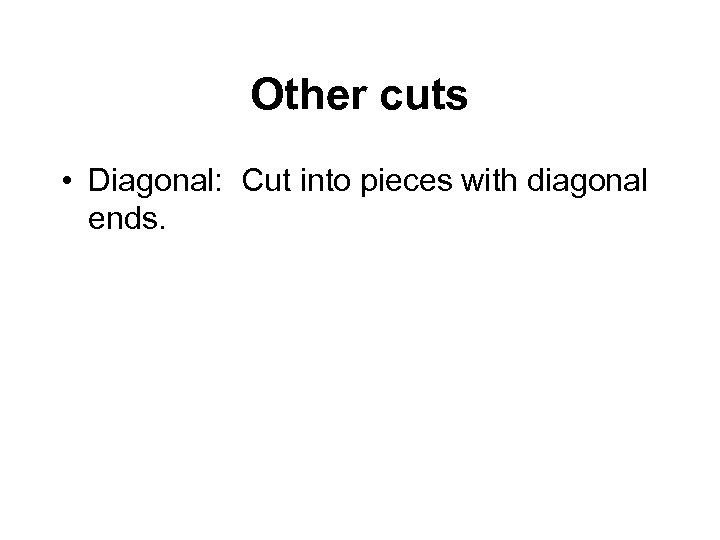Other cuts • Diagonal: Cut into pieces with diagonal ends.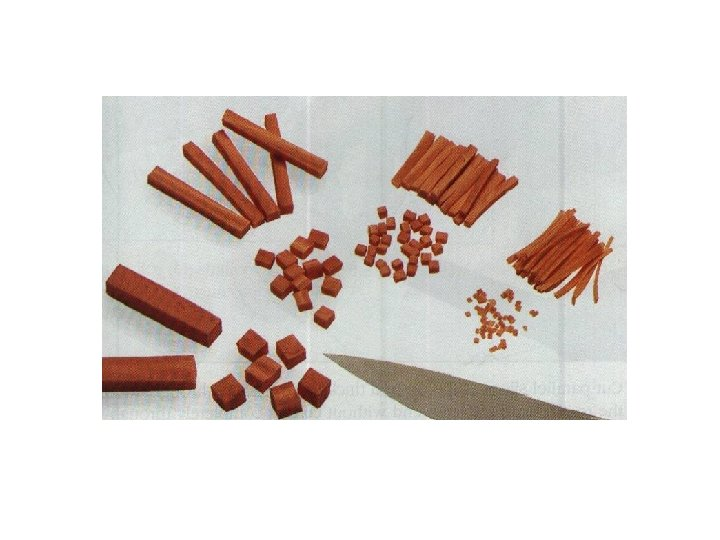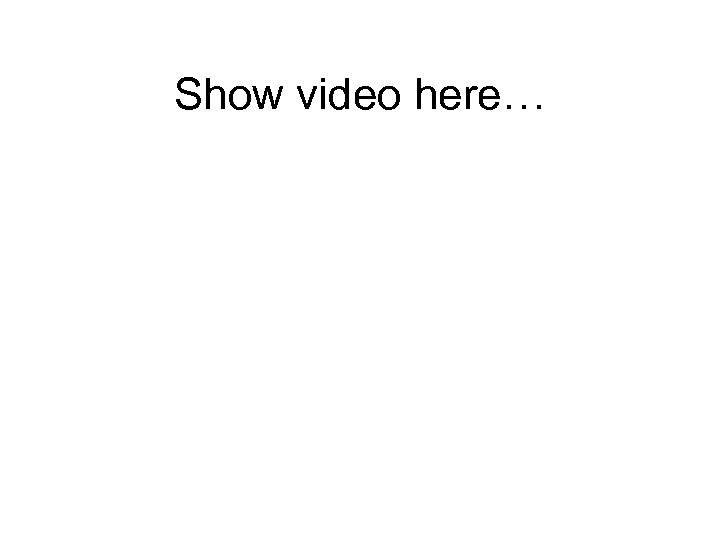Show video here…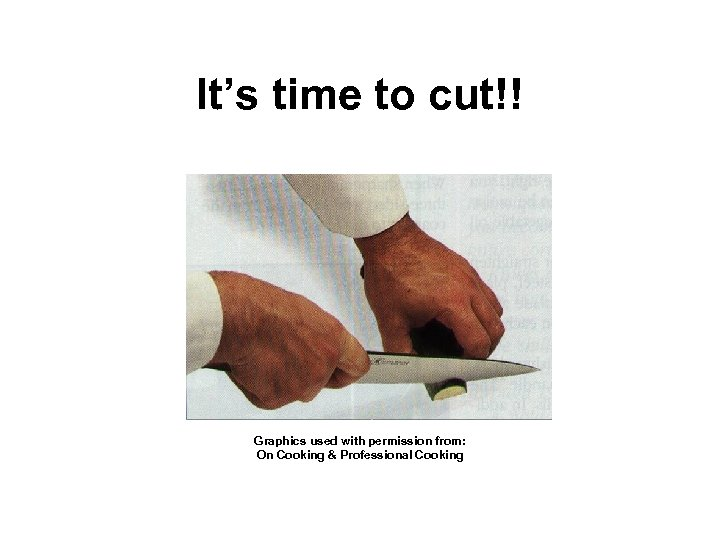It’s time to cut!! Graphics used with permission from: On Cooking & Professional Cooking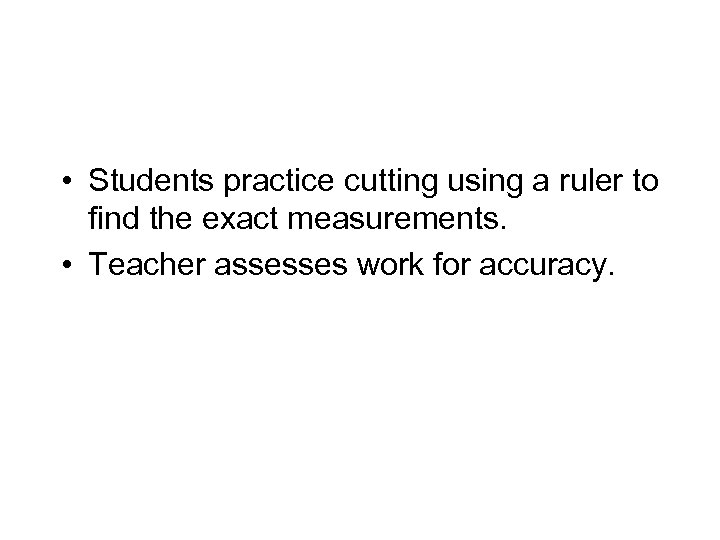• Students practice cutting using a ruler to find the exact measurements. • Teacher assesses work for accuracy.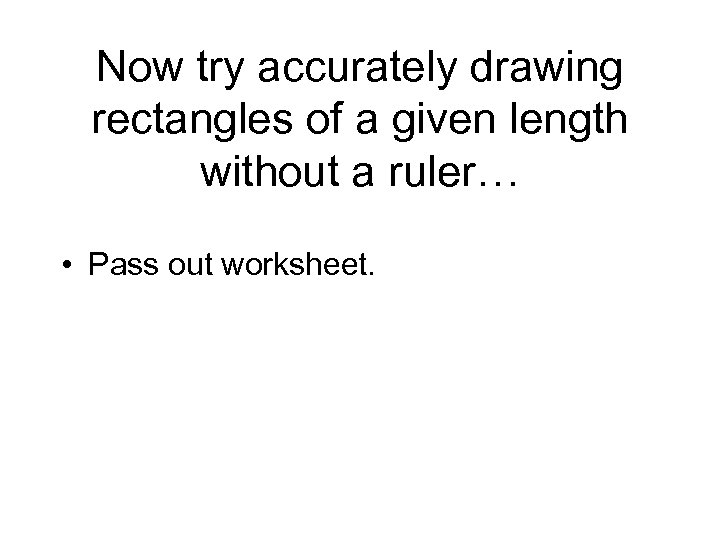Now try accurately drawing rectangles of a given length without a ruler… • Pass out worksheet.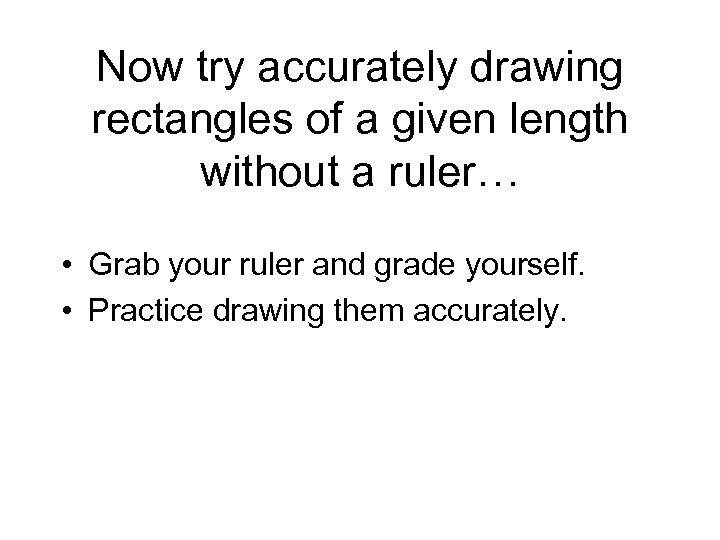Now try accurately drawing rectangles of a given length without a ruler… • Grab your ruler and grade yourself. • Practice drawing them accurately.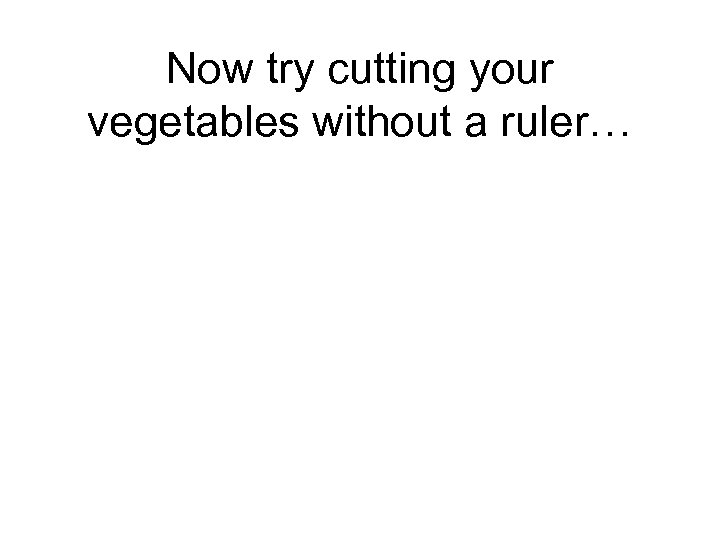Now try cutting your vegetables without a ruler…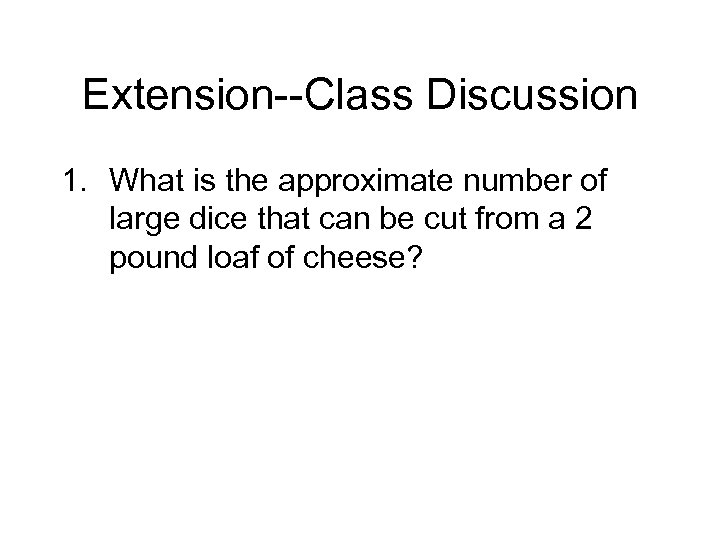Extension--Class Discussion 1. What is the approximate number of large dice that can be cut from a 2 pound loaf of cheese?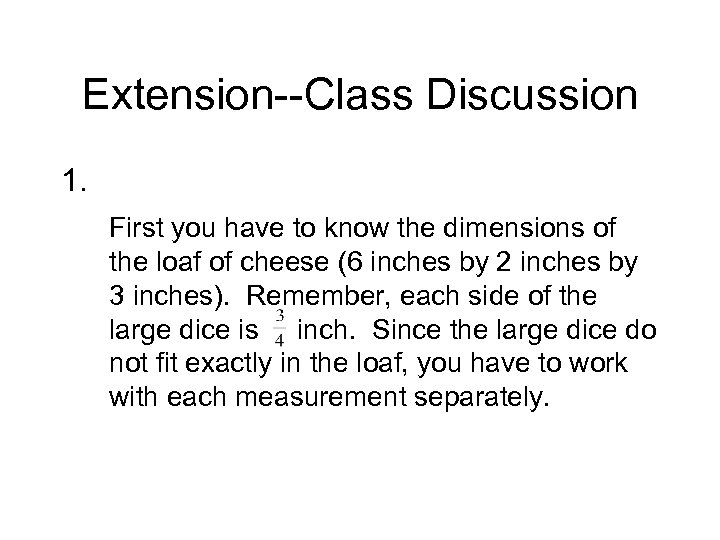Extension--Class Discussion 1. First you have to know the dimensions of the loaf of cheese (6 inches by 2 inches by 3 inches). Remember, each side of the large dice is inch. Since the large dice do not fit exactly in the loaf, you have to work with each measurement separately.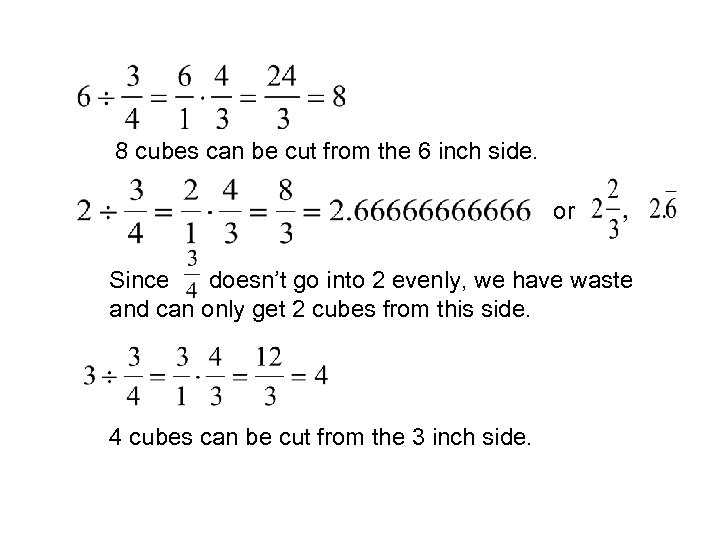8 cubes can be cut from the 6 inch side. or Since doesn’t go into 2 evenly, we have waste and can only get 2 cubes from this side. 4 cubes can be cut from the 3 inch side.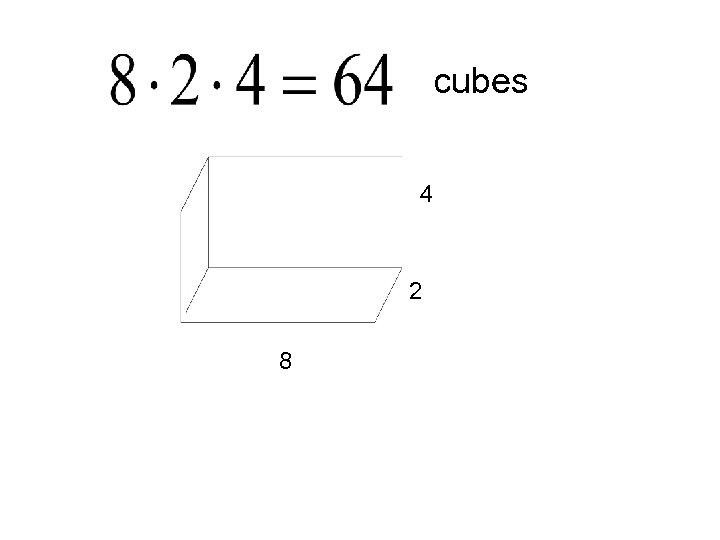cubes 4 2 8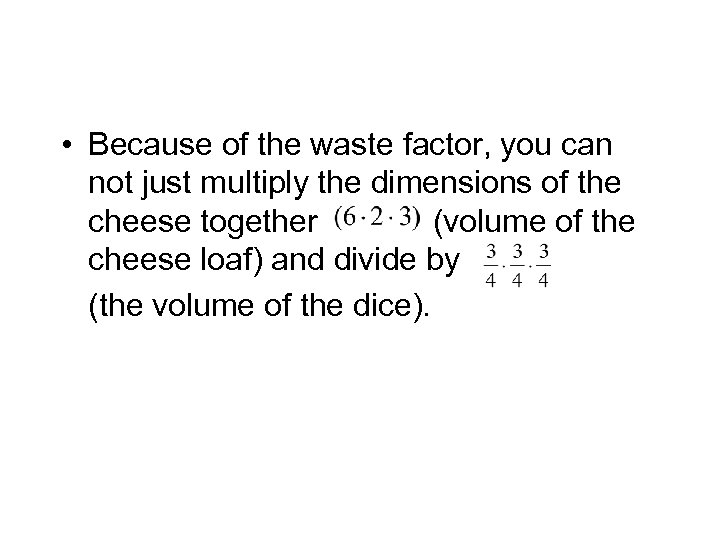• Because of the waste factor, you can not just multiply the dimensions of the cheese together (volume of the cheese loaf) and divide by (the volume of the dice).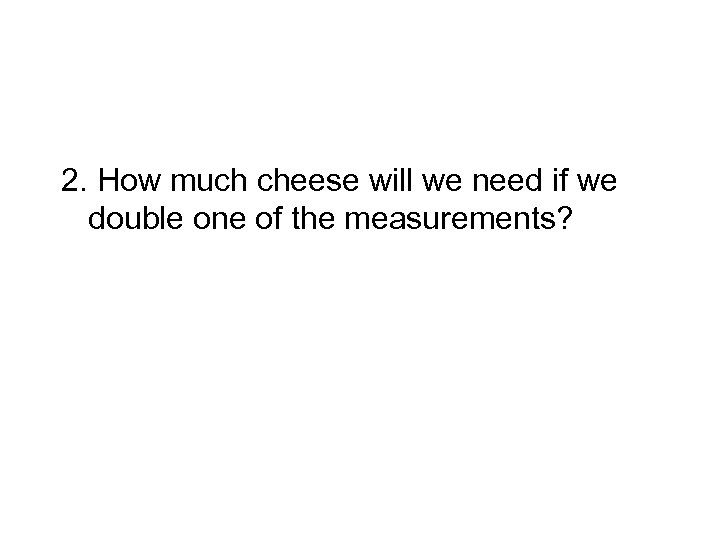2. How much cheese will we need if we double one of the measurements?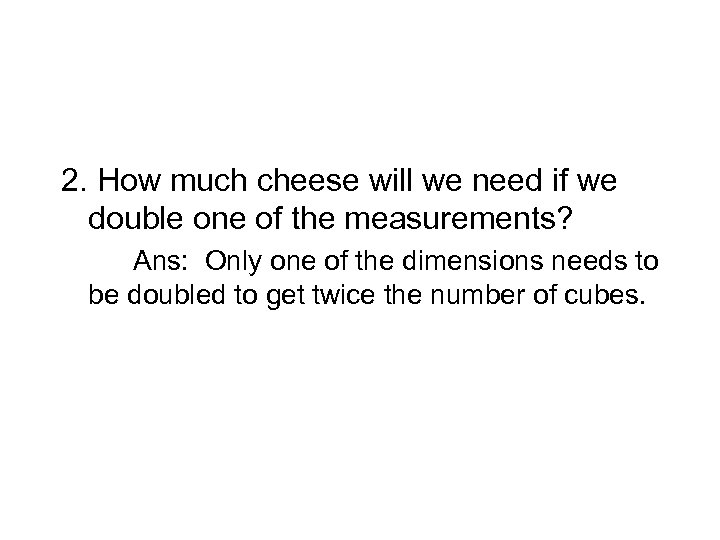2. How much cheese will we need if we double one of the measurements? Ans: Only one of the dimensions needs to be doubled to get twice the number of cubes.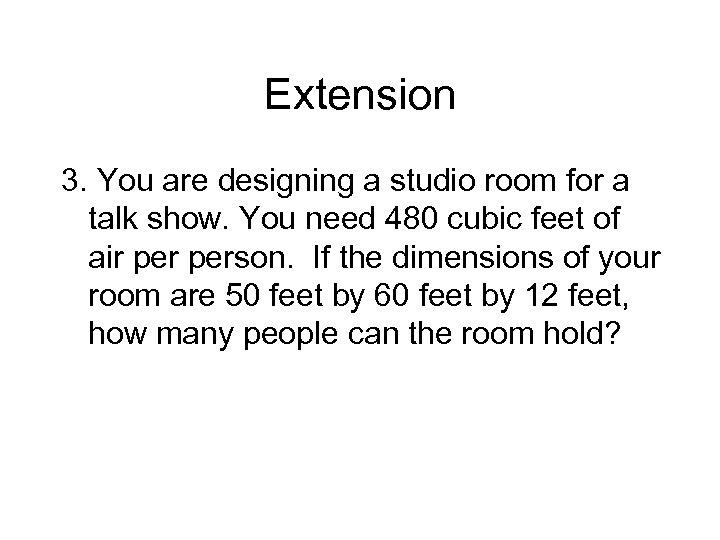Extension 3. You are designing a studio room for a talk show. You need 480 cubic feet of air person. If the dimensions of your room are 50 feet by 60 feet by 12 feet, how many people can the room hold?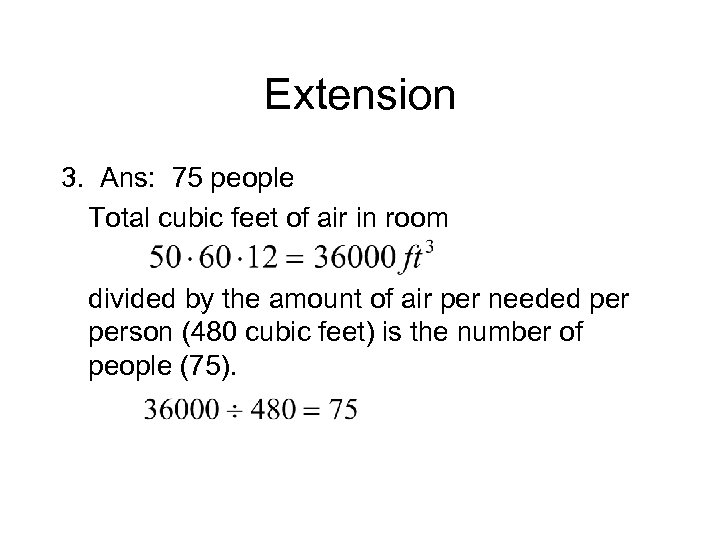Extension 3. Ans: 75 people Total cubic feet of air in room divided by the amount of air per needed person (480 cubic feet) is the number of people (75).